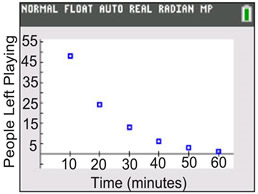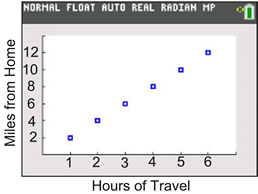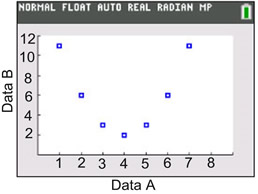Practice Fitting Functions Terms of Use    Contact Person: Donna Roberts1.  Participants are being eliminated every 10 minutes from a gaming competition. The data is shown in the chart at the right, with a calculator scatter plot below the chart.
a)
Examine the scatter plot. Which type of regression model will be the best fit for this data?
 Choose: linear regression exponential regression quadratic regression

b)
Which choice is the regression equation?
 Choose: y = 112.26(0.93)x y = -0.87x + 46.33 y = 0.03x2 - 2.65x + 70
 Time (minutes) People Left Playing 10 48 20 24 30 13 40 6 50 3 60 12.  Kevin is biking to visit his friend who lives in a nearby town. The data is shown in the chart at the right, with a calculator scatter plot below the chart.
a) Examine the scatter plot. Which type of regression model will be the best fit for this data?
 Choose: linear regression exponential regression quadratic regression

b)
Which choice is the regression equation?
 Choose: y = 1.80(1.41)x y = 2x + 2 y = 2x
 Hours of Travel Miles from Home 1 2 2 4 3 6 4 8 5 10 6 123.  Two data sets are being compared. The data is shown in the chart at the right, with a calculator scatter plot below the chart.
a)
Examine the scatter plot. Which type of regression model will be the best fit for this data?
 Choose: linear regression exponential regression quadratic regression

b)
Which choice is the regression equation?
 Choose: y = 5(1)x y = 0x + 6 y = x2 - 8x + 18
 Data A Data B 1 11 2 6 3 3 4 2 5 3 6 6 7 11